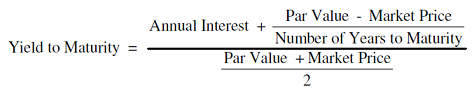## Sunday, June 14, 2015

### What is yield?

What exactly is  yield and how does it differ from coupon rate?
The term yield is used to describe the return on your investment as a percentage of your original investment.
Yield in the case of stocks
Yield is the ratio of annual dividends divided by the share price. If a stock can be expected to pay out Rs 1 as dividend over the next year and is currently trading at Rs 50, its dividend yield is 2%. Or, if the stock price drops to Rs 25, its dividend yield rises to 4%.
The yield can be calculated based on dividends paid over the past year or dividend expectations for the next.
Yield in the case of bonds
In the case of a bond, the yield refers to the annual return on an investment. The yield on a bond is based on both the purchase price of the bond and the interest promised – also known as the coupon payment.
Although a bond’s coupon rate is usually fixed, its price fluctuates continuously in response to changes in interest rates in the economy, demand for the instrument, time to maturity, and credit quality of that particular bond.
As a result, after bonds are issued, they trade at premiums or discounts to their face values until they mature and return to full face value.
Let’s say a bond’s face value is Rs 1,000 on which an investor can earn 5%. This means that the coupon is 5% and an investor who buys the bond and holds it till maturity will get Rs 50 every year over the tenure of the bond.
Now the price of the bond drops in the market to Rs 980. That means the current yield is Rs 50 divided by Rs 980 = 5.10%.
Later, the price of the bond rises to Rs 1,030. That means the current yield is Rs 50 divided by Rs 1,030 = 4.85%.
As the price of the bond fell, its yield increased.
Because yield is a function of price, changes in price result in bond yields moving in the opposite direction. There are two ways of looking at bond yields - current yield and yield to maturity.
Current Yield
This is  the annual return earned on the price paid for a bond. It is calculated by dividing the bond's coupon rate by its purchase price.
For example, let’s say a bond has a coupon rate of 6% on a face value of Rs 1,000. The interest earned would be Rs 60 in a year. That would produce a current yield of 6% (Rs 60/Rs 1,000).
When a bond is purchased at face value, the current yield is the same as the coupon rate. But let’s say the bond was purchased at a discount to face value – Rs 900. The current yield would be 6.6% (Rs 60/ Rs 900).
Yield to Maturity
This reflects the total return an investor receives by holding the bond until it matures. A bond’s yield to maturity, or YTM, reflects all of the interest payments from the time of purchase until maturity, including interest earned on interest.
The formula for calculating YTM is as follows.Let's work it out with an example: Par value (face value) = Rs 1,000 / Current market price = Rs 920 / Coupon rate = 10%, which means an annual coupon of Rs 100 / Time to maturity = 10 years.
Taking the above example and using the formula, the YTM would be calculated as follows:
YTM = Rs 100 + [(Rs 1,000-Rs 920)/10] / (Rs 1,000+Rs 920)/2
After solving the above equation, the YTM would be 11.25%.